﻿ Static and Modal Analysis and Optimization of Cross-Sectional Area of the Manipulator Arm

Static and Modal Analysis and Optimization of Cross-Sectional Area of the Manipulator Arm

Ján Vavro jr., Ján VavroOPEN ACCESSPEER-REVIEWED

Static and Modal Analysis and Optimization of Cross-Sectional Area of the Manipulator Arm

Ján Vavro jr.1, Ján Vavro1,1University of Alexander Dubček in Trenčín, Faculty of Industries Technologies, Púchov, Slovakia

Abstract

The given paper is focused on static analysis, modal analysis and optimization of the cross-sectional area of the loaded manipulator arm with the help of the computational modelling based on finite element method with utilization of Pro-Engineer and Cosmos M programs. From the aspect of designed object, the designer's main task is to incorporate such parameters which will be in harmony with the requirements of its practical application and which will also include its mass, shape, geometry, and some dynamic properties. The main aim of this paper is to enhance or optimize in-service part of machine while it is necessary to save the processed material and it is also important to find the best solutions not only in terms of the machine operation in the production process, but also in terms of an adequate material utilization and in terms of a suitable shape of the designed structure.

At a glance: Figures

123
Prev Next

• jr., Ján Vavro, and Ján Vavro. "Static and Modal Analysis and Optimization of Cross-Sectional Area of the Manipulator Arm." American Journal of Mechanical Engineering 1.7 (2013): 236-240.
• jr., J. V. , & Vavro, J. (2013). Static and Modal Analysis and Optimization of Cross-Sectional Area of the Manipulator Arm. American Journal of Mechanical Engineering, 1(7), 236-240.
• jr., Ján Vavro, and Ján Vavro. "Static and Modal Analysis and Optimization of Cross-Sectional Area of the Manipulator Arm." American Journal of Mechanical Engineering 1, no. 7 (2013): 236-240.

 Import into BibTeX Import into EndNote Import into RefMan Import into RefWorks

1. Introduction

Nowadays, the enhancement of the production process, safety conditions at, cost effectiveness, elimination of material waste as well as reliability are necessary to be considered because the optimisation and safety during the whole manufacturing process is closely connected with requirements where all of these mentioned factors has to be taken in account. The increasing requirements in relation to the improvement of the working approaches procedures can be observed in all fields of industries including rubber industry. From the aspect of safety and in-service effectiveness, the attention has to be paid to the devices and their operating parts and therefore, the main objective of the given paper is focused on dynamic and static analysis as well as optimization of conditions for the cross-sectional area of the manipulator K1 – K5 (Figure 1, 2) in the order to keep the requirements relating to safety and effectiveness. In relation to the rubber industry, the given device is used as modifying mould during the vulcanisation procedure relating to tyre manufacturing. The analyses and optimisation was performed for manipulator arm LA 425 (Figure 2) and it was done with reference to operating loading. Manipulator was designed by the help of the Pro/Engineer system. The geometry of the given manipulator was transformed by the Igs file to program for creation of finite–element model.

Calculation of arm LA 425 as a part of manipulator K1 - K5 was made on the basis of static loading 20 000 [N for the highest extension of the arm where the loading is the highest. Using the volume elements, the finite – element model (Figure 3) was made for manipulator arm LA 425 (Figure 2).

Arm is made of material 11 523.1.

Stability equations are solved in this way :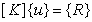(1)

Static analysis of the manipulator arm includes solution of the stability equations (1). System of equation is solved by Gaussian method of the elimination. This method includes positive definite symetric system of equation. The algorithm performs minimal operation number. There are not performed LTDL operations with zero elements.

After the calculation of the nodal point displacements, calculation of the loading of elements is made. The relation between deformation vector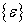and displacement are expressed by equation: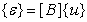(2)

where [B] is transformation of deformation – matrix of displacement. Loading vector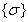represents a conjunction of the transformation deformation - matrix loading [D ] with the deformation vector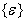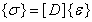(3)

Usage of (2) in (3), the relation between loading vector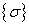and vector of nodal point displacements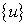can be obtained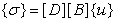(4)

Distribution of the stresses in arm of manipulator is given in MPa and it can be seen in the Figure 4 and detail of the distribution of stresses is shown in the Figure 5.

3. The Calculation of the Eigenfrequencies and Eigenshapes for Manipulator arm

Mathematical model can be expressed in form (5)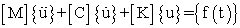(5)

where M is the mass matrix, C is the damping matrix, K is the stiffness matrix,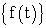is the time varying loading vector and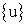,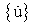,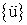are the displacement, velocity and acceleration vectors, respectively.

In relation to the calculation of the arm LA 425 of manipulator K1 - K5, the static loading was 4 000 kg. The computational model (Figure 2) representing the manipulator arm LA 425 (Figure 3) was created by help of the eightnodal volume elements.

Modal and frequency analysis were done according to known equation in the form: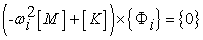(6)

where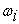is the i-th eigenfrequency,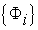is the eigenvector representing the nodal shape of the i-th eigenfrequency. We calculated eigenfrequencies and from the eigenfrequencies and on the basis of the eigenfrequencies, the frequency characteristics with the modal damping  = 0,001 was calculated The motion equation for the i-th eigenshape is in accordance with: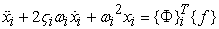(7)

This relationship is generalized problem of the eigenvalues where solution is made by the semi-automatic-space iterative method. This method is based on the idea of the inverse iteration conversion with several vectors at the same time.

In the Figure 6, we can see the first three shapes of the eigenfrequencies and in Table 1, there are the first 10 eigenfrequencies calculated by the help of the underspace method.

Table 1. The first ten eigenfrequenciesDownload asPowerPoint Slide

Veiw figureView current table in a new window

4. Optimization of Cross-sectional Area of the Manipulator Arm

In relation to the material saving, long service life, reliable and safe operation of mechanical equipment, nowadays strict requirements call for new approaches in putting various technical ideas into practice. It can be fulfilled only with the usage of suitable computing systems for fast and efficient study of the parameters which influence the static or dynamic characteristics of a machine in a greater or a lesser extent.

4.1. General Formulation of the Optimization Problem

In the first step, it is necessary to create correct physical machine model (static or dynamic). Second step is focused on the investigation and model description by the proper mathematic means – creation of the computational model (equations system, couplings conditions, etc.) The third and often most difficult procedure of optimum construction designing is connected with mathematical formulation of the optimization problem. It can be formulated as looking for extreme conditions (it is minimum value of extreme conditions) of the objective function: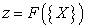(8)

Furthermore, it is based on additional (limiting) conditions which are given by irregularities which determinate admissible area: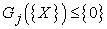(9)

where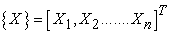is vector of designing (design) variables. Equations (8) and (9) contain the general non-linear functions of designing parameters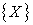. They belong to problems of mathematic programming which is often based on non-linearity. For research of global minimum, KuhnTucker obligatory conditions were used: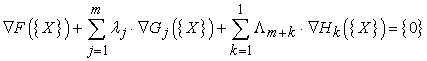(10)

Mathematic programming belongs to direct methods of optimization problems solution. In consequence of general non-linear character of objective function and limitation functions, equations (8), (9), (10), there will be non-linearity. In majority cases, this state can not be solved analytically. Then it is necessary to apply iterative methods, which allow computing vectors chain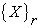(r = 1, 2.....) which converge to optimum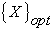. Direct equation application (10) in optimization process impacts on the problems with the limitations in non-equations form and therefore, there are other methods of non-linear programming used. The most common application in optimization of problems relates to methods of “zero” place value (comparator) and methods of “first” place value (gradient) but the second one mentioned methods are more effective. Their general disadvantage is that there is the problem to perform the analysis of sensitivity. Fundamentally, it deals with various modified iterative methods of type. General idea of these iterative approaches can be expressed by equation: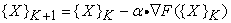(11)

Just like in the equation (10), the operator ∇ expresses gradient of objective function in the k-th iterative step for direction of progress optimum finding. It has been shown in practice that direct optimizing methods involve rather demanding computations which can be quite complicated from the technical aspect. However, there are also indirect methods that do not operate directly with the target function and they are used only for evaluation of the limitations (usually stresses). One of such methods is the “Fully stress design”, and we will utilize its basic principles and procedures hereinafter. The method is based on the idea of designing the structural variables in such a manner that the stresses in the entire structure will be at the permissible limit.

4.2. The method of “Fully Stress Design”

This method is used to find an optimum solution utilizing the overall loading capacity of the material at the given loading. The limiting conditions for the stresses may be written as follows: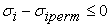(12)

where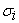isthe stress for the given point and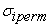is the maximum permitted stress. As an example, an optimum design of beam structures will be used with respect to its sectional characteristics.

4.3. Optimization of Sectional Parameters by Beam Elements

We consider duo node beam finite element with constant cross-section. In relation to this element, there are slide forces, axial forces, torque constant and elastic moments changing in a linear way between both ending nodal points. Maximum stress will be for one or other end of the beam, therefore points of calculated stresses will be there. Except this fact, we have define also position of points, for which we compute stresses in cross-sectional area. Final stresses will be calculated according to equations:

axial compound of normal stress: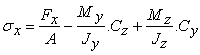(13)

slide stress from slide forces: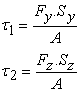(14)

slide stress from torque: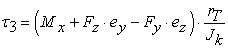(15)

where Fx, Fy, Fz are compounds of internal force in given cross-section, Mx, My, Mz are internal moments, A is cross-sectional surface, Jy and Jz are axial quadratic moments of persistence, Jk is cross-sectional torque moment, Sy and Sz are static moments of cross-sectional part dedicated by point area where we calculate the stress, cy and cz are point distances in section plane, in which we calculate stresses from cross-sectional centre of gravity, ey and ez are coordinates of eccentricity (distance between centre of gravity and slide centre), rT is effective radius of torsion. Equivalent stress is calculated according to HMH hypothesis (von Misses):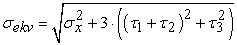(16)

Computational points for beam cross-section have to be defined for the sites where the extreme values can be expected. Furthermore, we have to define stiffness matrix of three-dimensional beam finite element and calculation of internal force parameters, from which the stresses in endpoint nodes will be assigned according to equations (13), (14), (15). If axis y, z will be central, then Sy = 0, Sz = 0, Dyz = 0 and we obtain known, in literature very often published, stiffness matrix of beam element. There are many approaches in optimization of beam cross-section. One of the approaches can be optimization of total height, width or both cross-sectional variables. The optimization modulus in Cosmos program can be used for this work.

4.4. Optimization of Cross-sectional Area of the Manipulator Arm

Optimization of the manipulator arm was done in program COSMOS 2.85 and the optimum designing of the model (Figure 2) led to optimization of the whole area of beam arm.

The cross-sectional area of arm for individual beam elements was changed with element width of arm and element height was constant. Stress limitation value was specified at σidov = 150 [MPa]. The arm was loaded by own weight and maximal (standard) force 20 000 N at one and second side of arm. Arm weight before optimization was 48.9 kg.. Stress distribution in manipulator arm before optimization is in the Figure 4. Stress distribution in manipulator arm after optimization is at Figure 7.

Optimization results for individual iterative steps are in the Figure 8.

As we can see from Figure 8, minimal arm weight was reached in the tenth iterative step. We reduced arm weight by 3,975 kg.

5. Conclusion

In relation to total manipulator arm loading, there is the influence of the third shape of eigenfrequency on manipulator arm vibration which causes vibration in the vertical direction.. The first and the second eigenshape represent vibration in horizontal direction and this vibration does not have any influence on operation of manipulator. The Finite Element Method enables to solve many complex practical tasks that, in the past, were almost insolvable. It cannot be generally concluded that some particular method is the best and that it always leads to finding the optimal solution. Each problem is peculiar in its own way due to its physical or mathematical model or due to the procedure used in the finding of the best solution. Therefore, during the looking for the effective procedure for the optimization of mechanically stressed structures, the following principles should be taken into account:

- special approach to the problem under the solution (static or dynamic analysis, linear or non-linear behaviour of the structure),

- the number of designed variables and their character,

- expected location of the optimum with regard to the limitations.

It is necessary to point out that designers cannot consider the static optimizing analysis as the final design before the dynamic analysis is carried out. The important point here is that the designer should take in account the influence of the changes of rigidity and mass of the structure upon its dynamic characteristics.

Acknowledgement

The work presented in this paper was supported by VEGA grant No. 1/0530/11.

References

  Azar, J., J.: Matrix Structural Analysis,Pergamon Press, New York, 1972.In article PubMed  Frankovský, P., Pástor, M.,Kenderová, M.: Automatization of Strain and Stress Fields Assessment Based on the Principles of reflection Photoelasticity.In: EAN 2012 Proceedings of the 50th annual conference on experimental stress analysis. , 2012.In article  Frankovský, P., Ostertag, O., Ostertagová, E.: Automation of experiments in photoelasticity. In:Procedia Engineering , 2012, No. 48, p. 153-157. ISSN 1877-7058.In article  Frankovský, P., Kostelníková, A., Šarga, P.: The use of strein-gage method and PhotoStress method in determining residual stresses of steel console. In: Metalurgia. Vol. 49, No 2 (2010), p.208-212, ISSN0543-5846.In article  Bocko, J., Trebuňa, F., Pástor, M., Frankovský, P.,: Application of Lie groups in the study of beam and plate vibrations.In: EAN 2010 ,48th International scientific conference,Velké Losiny, , 2010, p.19-26, ISBN 978-80-244-2533-7.In article